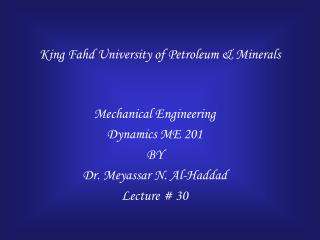DownloadDownload PresentationKing Fahd University of Petroleum & Minerals

# King Fahd University of Petroleum & Minerals

Télécharger la présentation## King Fahd University of Petroleum & Minerals

- - - - - - - - - - - - - - - - - - - - - - - - - - - E N D - - - - - - - - - - - - - - - - - - - - - - - - - - -
##### Presentation Transcript

1. King Fahd University of Petroleum & Minerals Mechanical Engineering Dynamics ME 201 BY Dr. Meyassar N. Al-Haddad Lecture # 30

2. CHAPTER 17 Planar Kinetics of a Rigid Body: Force and Acceleration

3. Objective • Moment of Inertia of a body • Parallel Axis Theorem • Radius of Gyration • Moment of Inertia of Composite Bodies

4. Moment and Angular Acceleration • When M  0, rigid body experiences angular acceleration • Relation between M and a is analogous to relation between F and a Mass = Resistance Moment of Inertia

5. Moment of Inertia • This mass analog is called the moment of inertia, I, of the object • r = moment arm • SI units are kg m2

6. Shell Element Disk Element

7. Example 17-1

8. L R2 R2 R a R b R R Moments of inertia for some common geometric solids L a b

9. Parallel Axis Theorem • The moment of inertia about any axis parallel to and at distance d away from the axis that passes through the centre of mass is: • Where • IG= moment of inertia for mass centre G • m = mass of the body • d = perpendicular distance between the parallel axes.

10. Radius of Gyration Frequently tabulated data related to moments of inertia will be presented in terms of radius of gyration.

11. Mass Center 10 Ib Example

12. Moment of Inertia of Composite bodies • Divide the composite area into simple body. • Compute the moment of inertia of each simple body about its centroidal axis from table. • Transfer each centroidal moment of inertia to a parallel reference axis • The sum of the moments of inertia for each simple body about the parallel reference axis is the moment of inertia of the composite body. • Any cutout area has must be assigned a negative moment; all others are considered positive.

13. Moment of inertia of a hollow cylinder • Moment of Inertia of a solid cylinder • A hollow cylinder I = 1/2 mR2 - = m2 M m1 R1 R2 I = 1/2 m1R12 - 1/2 m2R22 = 1/2 M (R12 - R22 )

14. Example 17-3

15. Example 17-4

16. Thank You